## Mercator's Projection

The Mercator projection was invented by Gerardus Mercator, a Flemish mapmaker.His name is a latinized version of Gerhard Kramer.

This is his famous world map of 1569.### A modern Mercator projection mapThe property of the Mercator projection map that made it useful to navigators is that it preserves angles. Lines of constant compass heading (called rhumb lines by sailors) are straight lines on this map. Say you're in Vancouver and want to get to Honolulu, Hawaii. You don't have GPS, just a compass.Just draw a straight line on the map between where you are and where you want to go, and measure the angle (almost exactly 45 degrees in this case). So (after a small detour around Vancouver Island) you head southwest, and off you go. If you keep heading in this direction, you ought to get there.

Earlier maps were drawn on a simple grid: each degree of latitude or longitude is the same size. This method wouldn't work there: rhumb lines would be curves. Mercator knew that to give his map this desirable property, he had to make the lines of latitude farther apart as you go away from the equator. But he didn't say exactly how he did it. He almost certainly didn't have a formula, but might have done it using approximate measurements on a globe. He just said

It is for these reasons that we have progressively increased the degrees of latitude towards each pole in proportion to the lengthening of the parallels with reference to the equator.

### How NOT to do itMany people have an incorrect idea of how Mercator's projection works. This seems to be commonly taught in high schools. The idea is to imagine a cylinder with the globe inside, and light projected from the centre through the globe onto the cylinder, as in the picture here. Then unroll the cylinder. This cylindrical projection is NOT Mercator's projection.

If you want a physical model of Mercator's projection, let the globe be a spherical balloon that is blown up inside the cylinder, and sticks to the cylinder when it comes into contact with it.

### How to do itIn 1599 the English mathematician Edward Wright explained the mathematics of exactly how Mercator's projection should be done. Let's say that the distance on Mercator's map from the equator to the parallel at latitudeis, in units where the equator has lengthon the map.

Here is a picture of the Earth with the top sliced off at latitude. The equator is a circle with radius(the radius of the Earth) and circumference, and the parallel at latitudeis a circle of radiusand circumference. But on the map the parallels are all the same length. So the parallel at latitudeis stretched by a factor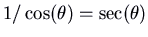. Now imagine a very small square piece of land at latitude, with sides of lengthoriented north-south and east-west. To go from its northeast corner to its southwest corner, you travel southwest. In order for Mercator's projection to work, this has to be true for its image on the map as well: so its image on Mercator's map must be a square. It takes up a fraction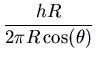of the parallel at latitude, so its width on the map is. So its height on the map must also be. Now really the piece of land isn't all at exactly the same latitude, it goes fromtosay, where the side is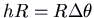, i.e.. And the formula for the height on the map is approximate. So the height on the map, which is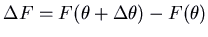, is approximately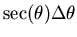. Thus. In the limit as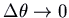, we get. Thusis an antiderivative of. Taking the equator as height 0, so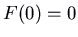, we can write it as a definite integral:That's not quite the language Wright uses (this was before the invention of calculus), but it amounts to the same thing.

Well, now the problem is to do the integration. Wright didn't know how. In fact, he didn't know about logarithms yet, and as we'll see we need logarithms to write down the answer. So what Wright did was to do the integration approximately, using sums (like the left or right sums we did earlier) with an interval of 1 minute of arc = 1/60 degree, for all latitudes up to 75 degrees. It must have taken a lot of effort back in those days. His table was published, and was quite useful for mapmakers.

In 1614 John Napier invented logarithms, and they were soon found to be quite useful in calculations. So useful that not only tables of logarithms, but tables of logarithms of trigonometric functions, were published, including in 1620 a table of logarithms of tangents.

In the 1640's a man by the name of Henry Bond happened to be looking at two tables at the same time: Wright's table ofand a table of logarithms of tangents. He noticed a coincidence:seemed to be the same as. He published this as a conjecture in 1645:Nowadays, all you would have to do to verify this is note that they agree at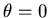, differentiate, and use a few trigonometric identities: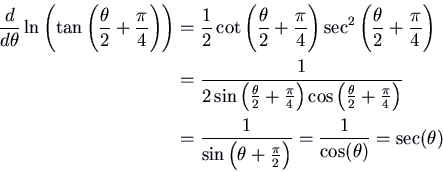But this was still before Calculus, so they couldn't do it that way. The problem actually became one of the famous open problems of the mid-17th century. Nicolaus Mercator (no relation to Gerardus) actually offered a prize for the solution. The problem was eventually solved in 1668 by James Gregory, but his proof was extremely complicated. A more intelligible proof was published by Isaac Barrow in 1670.

Robert Israel
2003-01-20